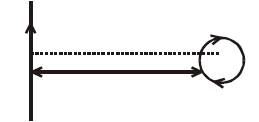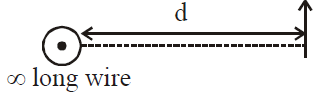# Q. An infinitely long current carrying wire and a small current carrying loop are in the plane of the paper as shown. The radius of the loop is a and distance of its centre from the wire is d (d > > a). If the loop applies a force F on the wire then :JEE Main JEE Main 2019

Solution:

## Eqvilent dipole of given loop $F =m . \frac{dB}{dr}$ Now $\frac{dB}{dx} = \frac{d}{dx} \left(\frac{\mu_{0}I}{2\pi x}\right)$ $\propto\frac{1}{x^{2}}$ $\Rightarrow \text{So} F \propto \frac{M}{x^{2}} \left[\because M = NIA\right]$ $\therefore F \propto\frac{a^{2}}{d^{2}}$You must select option to get answer and solution

## 1. The increase in pressure required to decrease the 200 L volume of a liquid by 0.004% (in kPa) is (Bulk modulus of the liquid ==2100 MPa)

Maharashtra CET 2006 Mechanical Properties Of Solids

## 2. To draw maximum current from a combination of cells, how should the cells be grouped?

AFMC 2006 Current Electricity

AFMC 2010 Atoms

NEET 2019 Waves

## 5. The period of revolution of planet A around the sun is 8 times that of B. The distance of A from the sun is how many times greater than that of B from the sun?

AIPMT 1997 Gravitation

## 6. Two discs of same moment of inertia rotating about their regular axis passing through centre and perpendicular to the plane of disc with angular velocities $\omega_1$ and $\omega_2$ . They are brought into contact face to face coinciding the axis of rotation. The expression for loss of energy during this process is:-

NEET 2017 System Of Particles And Rotational Motion

## 7. The temperature of 100 g of water is to be raised from 24°C to 90°C by adding steam to it. The mass of the steam required for this purpose is

JIPMER 2015 Thermal Properties Of Matter

## 8. The hot wire ammeter measures

Rajasthan PMT 2004 Alternating Current

## 9. The diameter of a flywheel is increased by 1%. Increase in its moment of inertia about the central axis is

JIPMER 2015 System Of Particles And Rotational Motion

## 10. A body of mass 4 kg is accelerated upon by a constant force, travels a distance of 5 m in the first second and a distance of 2 m in the third second. The force acting on the body is

KCET 2008 Laws Of Motion